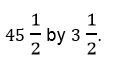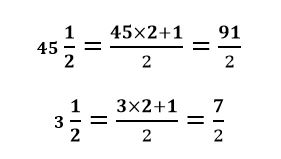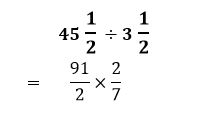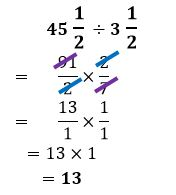# How to divide fractions with mixed numbers

Want to find out how to divide fractions with mixed numbers? We've provided a step-by-step guide of how to divide fractions with mixed numbers to teach you everything you need to know.## Step 1: Change the mixed numbers into an improper fraction

Multiply the whole number by the denominator and add the numerator to find the new numerator of the improper fraction. So given 5/12 ÷ 2 7/24, we can change the mixed number to and improper fraction.

## Step 2: Find the reciprocal of the divisor

To determine the reciprocal of a fraction, we switch the values of the numerator and denominator. So, for our given example, we have

## Step 3: Find the quotient

To find the quotient, we now multiply the dividend and the reciprocal to find the quotient of the fraction and mixed number.

## Step 4: Simplify the factors

Cancel out common factors shared between a numerator and denominator to simplify and multiply the remaining factors to find the quotient.

## Examples of dividing fractions with mixed numbers

Q) Anna has 45 1/2 inches of string and plans to cut them in to tinier pieces with a length of 3 1/2 inches. How many pieces of strings will she be able to cut?

To find the number of tinier pieces, we divide:Change both 45 1/2 and 3 1/2 into an improper fraction.Find the divisor’s reciprocal and hence, we have 2/7 .

Multiply 91/2 by the reciprocal to find the quotient.Cancel out common factors and multiply the remaining ones to find the quotient.This means that Anna will be able to cut a total of thirteen pieces.

#### Join today

The fastest way to practice

Unlock our complete testing platform and improve faster that ever.